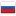The sum of the k-th powers of the first n positive integers – easy algorithm

Revision en1, by SirRembocodina, 2023-02-28 05:55:08

Suppose you want to know this sum for some n and k:Here are the well known formulas for the first several k:But suppose you forgot them. What to do? Luckily, there is an easy algorithm to generate those formulas.

First of all, let's prove a theorem.

### Theorem

Suppose for every integer non-negative n:where f and g are polynoms. Then for some constant c:### Proof

For every positive integer n:These two polynoms are equal in an infinite number of points, which means that they are identical. Which allows us to say:### Application

Let's say we want to find the formula for the sum of squares. Then using our theorem we can create such an algorithm:Now let's run the same algorithm to find the formula for the sum of cubes:#### History

RevisionsRev. Lang. By When Δ Comment
ru5SirRembocodina 2023-02-28 05:59:12 1290
en1SirRembocodina 2023-02-28 05:55:08 1446 Initial revision for English translation
ru4SirRembocodina 2023-02-28 05:54:39 0 (опубликовано)
ru3SirRembocodina 2023-02-28 05:47:24 20 Мелкая правка: 'noms. Then:\n\n![ ](' -> 'noms. Then for some constant c:\n\n![ ]('
ru2SirRembocodina 2023-02-28 05:45:38 833
ru1SirRembocodina 2023-02-28 05:34:18 859 Первая редакция (сохранено в черновиках)